# Medians and sides

Determine the size of a triangle KLM and the size of the medians in the triangle. K=(-5; -6), L=(7; -2), M=(5; 6).

Correct result:

k =  8.2462
l =  15.6205
m =  12.6491
t1 =  13.6015
t2 =  7.2801
t3 =  10.7703

#### Solution:

${t}_{2}=\sqrt{\left({x}_{1}-{b}_{0}{\right)}^{2}+\left({y}_{1}-{b}_{1}{\right)}^{2}}=\sqrt{\left(7-0{\right)}^{2}+\left(\left(-2\right)-0{\right)}^{2}}=\sqrt{53}=7.2801$

Try calculation via our triangle calculator.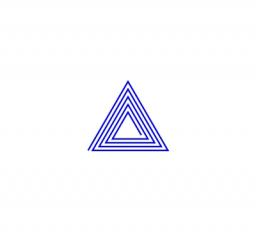We would be pleased if you find an error in the word problem, spelling mistakes, or inaccuracies and send it to us. Thank you!Tips to related online calculators
Do you want to convert length units?
Pythagorean theorem is the base for the right triangle calculator.

#### You need to know the following knowledge to solve this word math problem:

We encourage you to watch this tutorial video on this math problem:

## Next similar math problems:

• KLM triangleFind the length of the sides of the triangle KLM if m = 5cm height to m = 4.5 cm and size MKL angle is 70 degrees.
• Medians in triangle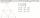Median of isosceles triangle has a length 3 cm. Determine the length of its sides if its perimeter is 16 cm.
• Medians and sidesTriangle ABC in the plane Oxy; are the coordinates of the points: A = 2.7 B = -4.3 C-6-1 Try calculate lengths of all medians and all sides.
• Right triangle from axesA line segment has its ends on the coordinate axes and forms with them a triangle of area equal to 36 square units. The segment passes through the point ( 5,2). What is the slope of the line segment?
• Center of line segmentCalculate the distance of the point X [1,3] from the center of the line segment x = 2-6t, y = 1-4t ; t is .
• Segment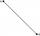Calculate the segment AB's length if the coordinates of the end vertices are A[10, -4] and B[5, 5].
• Medians in right triangleIt is given a right triangle, angle C is 90 degrees. I know it medians t1 = 8 cm and median t2 = 12 cm. .. How to calculate the length of the sides?
• Three points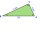Three points K (-3; 2), L (-1; 4), M (3, -4) are given. Find out: (a) whether the triangle KLM is right b) calculate the length of the line to the k side c) write the coordinates of the vector LM d) write the directional form of the KM side e) write the d
• MediansCalculate the sides of a right triangle if the length of the medians to the legs are ta = 21 cm and tb=12 cm.
• Three pointsThree points A (-3;-5) B (9;-10) and C (2;k) . AB=AC What is value of k?
• Vertices of a right triangleShow that the points D(2,1), E(4,0), F(5,7) are vertices of a right triangle.
• Triangle midpoints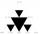Determine coordinates of triangle ABC vertices if we know tirangle sides midpoints SAB [0;3] SBC [1;6] SAC [4;5], its sides AB, BC, AC.
• Triangle ABCIn a triangle ABC with the side BC of length 2 cm The middle point of AB. Points L and M split AC side into three equal lines. KLM is isosceles triangle with a right angle at the point K. Determine the lengths of the sides AB, AC triangle ABC.
• Three altitudes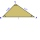A triangle with altitudes 4; 5, and 6 cm is given. Calculate the lengths of all medians and all sides in a triangle.
• CoordinatesDetermine the coordinates of the vertices and the content of the parallelogram, the two sides of which lie on the lines 8x + 3y + 1 = 0, 2x + y-1 = 0 and the diagonal on the line 3x + 2y + 3 = 0
• Coordinates of a centroindLet’s A = [3, 2, 0], B = [1, -2, 4] and C = [1, 1, 1] be 3 points in space. Calculate the coordinates of the centroid of △ABC (the intersection of the medians).
• Medians of isosceles triangleThe isosceles triangle has a base ABC |AB| = 16 cm and 10 cm long arm. What are the length of medians?